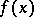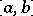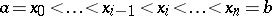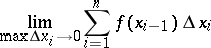# Cauchy integral

(diff) ← Older revision | Latest revision (diff) | Newer revision → (diff)

A Cauchy integral is a definite integral of a continuous function of one real variable. Letbe a continuous function on an intervaland let,,. The limitis called the definite integral in Cauchy's sense ofoverand is denoted byThe Cauchy integral is a particular case of the Riemann integral. The definition is due to A.L. Cauchy .

## Contents

How to Cite This Entry:
Cauchy integral. Encyclopedia of Mathematics. URL: http://encyclopediaofmath.org/index.php?title=Cauchy_integral&oldid=13156
This article was adapted from an original article by E.D. Solomentsev (originator), which appeared in Encyclopedia of Mathematics - ISBN 1402006098. See original article